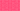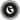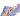PricingResources

# Sharpe Ratio: what is it and how to calculate itWritten by

An investment is deemed good if it offers higher returns but carries minimal risks. This should be the driving logic when picking assets to invest in. Sharpe ratio is one of the several ways to calculate the potential rewards of investing in an asset against its risks. Keep reading to know more about the meaning of the Sharpe Ratio and how to calculate it here.

## Introduction to the Sharpe Ratio

Developed by William Sharpe, a Nobel laureate economist, the Sharpe Ratio is used to calculate the risk-adjusted returns of a particular investment.

As mentioned above, investments are better judged when we factor in the inherent risks involved in them. Returns generated per unit of risk taken is a better metric for measuring how sound an investment is. But the question arises, how do you make such calculations?

The Sharpe Ratio Formula offers a simple method to help investors make these calculations. The formula looks like this:

Technically, we can represent this as: Sharpe Ratio = (Rp​−Rf)​ / σp​

Where:

• Rp = Average Returns of the Investment/Portfolio that we are considering.

• Rf = Returns of a Risk-free Investment.

• Sp= Standard Deviation of the Portfolio/Investment

In understanding this formula, there’s a need to explain the terms involved.

## Meaning of Terms

• Average Returns of the Investment/Portfolio: This refers to the average expected returns from the investment.

• Returns of a Risk-free Investment: The Risk-free Investment refers to an investment that is (almost) completely devoid of risk. As seen in the formula above, returns of a risk free investment are typically subtracted from the average returns of total investments. The purpose is to arrive at the net-returns that the investment actually generated.

The "risk-free investment" that is usually referenced is government treasuries, because they normally are expected to be devoid of any risks at all.

• Standard Deviation: The Standard Deviation of an investment tells us the amount by which the particular investment can go up or down, i.e. the volatility of the investment. In essence, the Standard Deviation refers to the risk involved with the investment.

## Sharpe Ratio Example

Here, we show a Sharpe Ratio calculation example to explain how it works.

Our desired investment is the stock of ABC Corp Plc. The stock has returned an average of 15% annually over the past five years.

The risk-free investment is the UK Treasury Bill which has an interest rate of 0.4%.

The standard deviation (volatility) of ABC Plc is put at 20%.

The Sharpe Ratio calculation = (15% - 0.3%) / 20%= 0.73.

## Uses of the Sharpe Ratio

The information derived from the Sharpe Ratio calculation can be used for various purposes:

### To compare investments

Helping to make objective comparison of assets for investment is one of the primary applications of the Sharpe Ratio.

Resources for investing in assets are finite, but assets to invest in are not. So investors are usually in a dilemma as to what investments they should commit to. The Sharpe Ratio can help you decide.

If after doing your Sharpe Ratio calculation, you discover that Stock B has a higher Sharpe Ratio than Stock A, then you may want to go for Stock B.

### To inform investments

When investors are confronted with the choice of making an investment, the Sharpe Ratio calculation can help decide if they should commit to such investments. By comparing the effective Sharpe Ratio of the investment being considered and, say, a risk-free investment like the Treasury Bills, an investor can make informed decisions. In essence, if the Sharpe Ratio of an investment is 2% and Treasuries return 4%, it may be better to go for Treasuries, considering the fact that the investor will encounter almost zero risk.

### Portfolio optimisation

Sharpe Ratio portfolio optimization is one of the most effective applications of the term. By comparing Sharpe Ratios of various investments, investors can determine which should be part of the portfolio.

## Sharpe Ratio Limitations

Should an investor base all investment decisions on the Sharpe Ratio calculation? Absolutely not. The fact that Tesla's stock has a higher Standard Deviation (that is, higher risk) and lower Sharpe Ratio than another stock does not make that other stock worthier for investing than Tesla.

There are many other considerations that investors should look into - such as the fundamentals of the particular investments.

That said, it is important to note that there is no single figure as to what a good Sharpe Ratio is. Whether the Sharpe Ratio of a particular investment is good can only be determined when we look at the Sharpe Ratio of another investment, amongst other factors.

## We can help

GoCardless helps you automate payment collection, cutting down on the amount of admin your team needs to deal with when chasing invoices. Find out how GoCardless can help you with ad hoc payments or recurring payments.Contact sales

Sales

Support